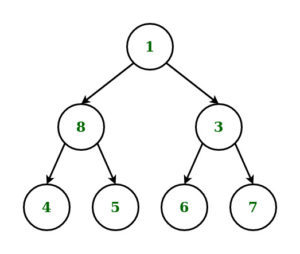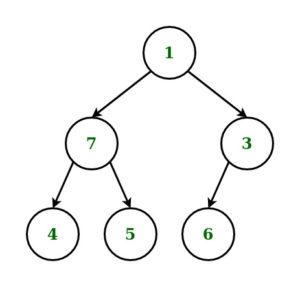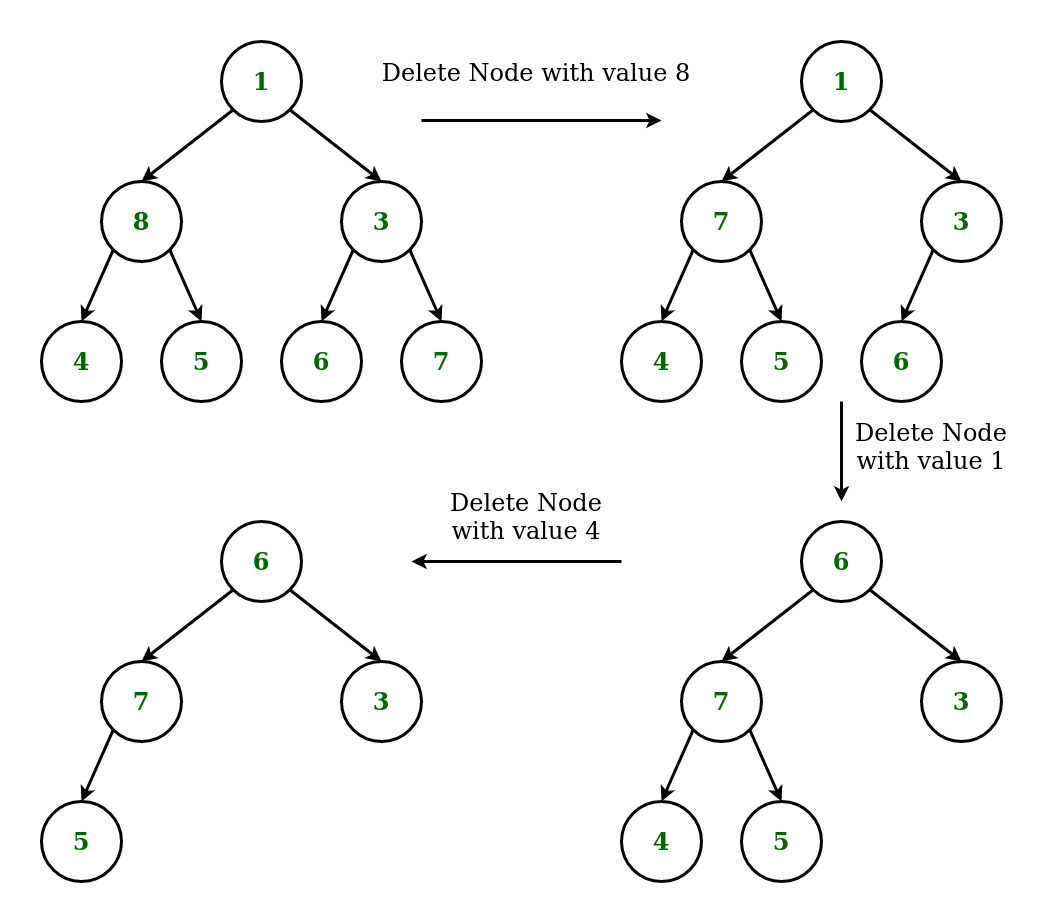# Deletion of a given node K in a Binary Tree using Level Order Traversal

Given a binary tree and a node K, the task is to delete the node K from it by making sure that tree shrinks from the bottom (i.e. the deleted node is replaced by bottom-most and rightmost node) using Level Order Traversal.

Examples:

Input: K = 8, Tree =Output:Explanation:

Approach:

• Start searching from the root, the address of node which is to be deleted by traversing in level order-wise.
• Continue Traversing Tree to find the deepest and rightmost node in level order wise to find the deepest and the rightmost node.
• If the node to delete is different from rightmost deepest node, then replace the node to be deleted with righmost deepest node and delete the later node
• If the node to delete is same as rightmost deepest node, then simply delete the node.

For example: Consider the Example 1 from aboveBelow is the implementation of the above approach.

## C++

 `// C++ implementation to delete a node ` `// in Binary Tree using Level Order Traversal ` ` `  `#include ` `using` `namespace` `std; ` ` `  `// Structure of Binary Tree ` `struct` `node { ` `    ``int` `data; ` `    ``node* left; ` `    ``node* right; ` `}; ` ` `  `// Function to create new node ` `// of a Binary Tree. ` `node* newnode(``int` `d) ` `{ ` `    ``node* temp = ``new` `node; ` `    ``temp->data = d; ` `    ``temp->left = temp->right = NULL; ` `    ``return` `temp; ` `} ` ` `  `// Function for Level Order ` `// Traversal of Binary Tree ` `void` `levelorder(node* curr) ` `{ ` ` `  `    ``// Queue to store the nodes ` `    ``// in a level ` `    ``queue q; ` `    ``if` `(curr) ` `        ``q.push(curr); ` ` `  `    ``// Loop to Traverse the Binary ` `    ``// Tree in Level Order ` `    ``while` `(!q.empty()) { ` `        ``curr = q.front(); ` `        ``q.pop(); ` `        ``cout << curr->data << ``" "``; ` `        ``if` `(curr->left) ` `            ``q.push(curr->left); ` `        ``if` `(curr->right) ` `            ``q.push(curr->right); ` `    ``} ` `} ` ` `  `// Function to Delete the deepest ` `// right-most node of the Binary Tree ` `void` `deletedeepest(node* root, ` `                   ``node* temp) ` `{ ` `    ``queue q; ` `    ``q.push(root); ` ` `  `    ``// Loop to Traverse Binary Tree ` `    ``// in level order and delete Node ` `    ``while` `(!q.empty()) { ` `        ``node* T = q.front(); ` `        ``q.pop(); ` `        ``if` `(T->right == temp) { ` `            ``T->right = NULL; ` `            ``delete` `(temp); ` `            ``return``; ` `        ``} ` `        ``else` `            ``q.push(T->right); ` `        ``if` `(T->left == temp) { ` `            ``T->left = NULL; ` `            ``delete` `(temp); ` `            ``return``; ` `        ``} ` `        ``else` `            ``q.push(T->left); ` `    ``} ` `} ` ` `  `// Function to Delete Node ` `// in Binary Tree ` `node* deletenode(node* root, ``int` `data) ` `{ ` `    ``if` `(root == NULL) ` `        ``return` `NULL; ` ` `  `    ``// Condition if the Root node ` `    ``// is a leaf node. ` `    ``if` `(root->left == NULL ` `        ``&& root->right == NULL) { ` `        ``if` `(root->data == data) { ` `            ``return` `NULL; ` `        ``} ` `        ``else` `            ``return` `root; ` `    ``} ` `    ``queue q; ` `    ``q.push(root); ` `    ``node* temp = NULL; ` `    ``node* nodetodelete = NULL; ` ` `  `    ``// Loop to Traverse Tree in ` `    ``// Level Order and find the ` `    ``// Rightmost Deepest Node of the ` `    ``// Binary Tree and Node to be Deleted ` `    ``while` `(!q.empty()) { ` `        ``temp = q.front(); ` `        ``q.pop(); ` `        ``if` `(temp->data == data) { ` `            ``nodetodelete = temp; ` `        ``} ` `        ``if` `(temp->left) { ` `            ``q.push(temp->left); ` `        ``} ` `        ``if` `(temp->right) { ` `            ``q.push(temp->right); ` `        ``} ` `    ``} ` ` `  `    ``// if node to be deleted is not found ` `    ``if` `(nodetodelete != NULL) { ` ` `  `        ``// Condition to check if node to delete ` `        ``// is not same as node to replace ` `        ``if` `(temp != nodetodelete) { ` `            ``int` `t = temp->data; ` `            ``deletedeepest(root, temp); ` `            ``nodetodelete->data = t; ` `        ``} ` ` `  `        ``// if node to delete is also ` `        ``// rightmost deepest node ` `        ``else` `{ ` `            ``deletedeepest(root, temp); ` `        ``} ` `    ``} ` `    ``return` `root; ` `} ` ` `  `// Driver Code ` `int` `main() ` `{ ` ` `  `    ``// Construction of Tree ` `    ``node* root = newnode(1); ` `    ``root->left = newnode(8); ` `    ``root->right = newnode(3); ` `    ``root->left->left = newnode(4); ` `    ``root->left->right = newnode(5); ` `    ``root->right->left = newnode(6); ` `    ``root->right->right = newnode(7); ` ` `  `    ``cout << ``"Original Tree: "``; ` `    ``levelorder(root); ` ` `  `    ``// Deleting node with key 8 ` `    ``root = deletenode(root, 8); ` `    ``cout << endl; ` `    ``cout << ``"Deleting node with key 8: "``; ` `    ``levelorder(root); ` ` `  `    ``// Deleting node with key 1 ` `    ``root = deletenode(root, 1); ` `    ``cout << endl; ` `    ``cout << ``"Deleting node with key 1: "``; ` `    ``levelorder(root); ` ` `  `    ``// Deleting node with key 4 ` `    ``root = deletenode(root, 4); ` `    ``cout << endl; ` `    ``cout << ``"Deleting node with key 4: "``; ` `    ``levelorder(root); ` `} `

Output:

```Original Tree: 1 8 3 4 5 6 7
Deleting node with key 8: 1 7 3 4 5 6
Deleting node with key 1: 6 7 3 4 5
Deleting node with key 4: 6 7 3 5
```

Don’t stop now and take your learning to the next level. Learn all the important concepts of Data Structures and Algorithms with the help of the most trusted course: DSA Self Paced. Become industry ready at a student-friendly price.

My Personal Notes arrow_drop_upCheck out this Author's contributed articles.

If you like GeeksforGeeks and would like to contribute, you can also write an article using contribute.geeksforgeeks.org or mail your article to contribute@geeksforgeeks.org. See your article appearing on the GeeksforGeeks main page and help other Geeks.

Please Improve this article if you find anything incorrect by clicking on the "Improve Article" button below.

Article Tags :
Practice Tags :

2

Please write to us at contribute@geeksforgeeks.org to report any issue with the above content.